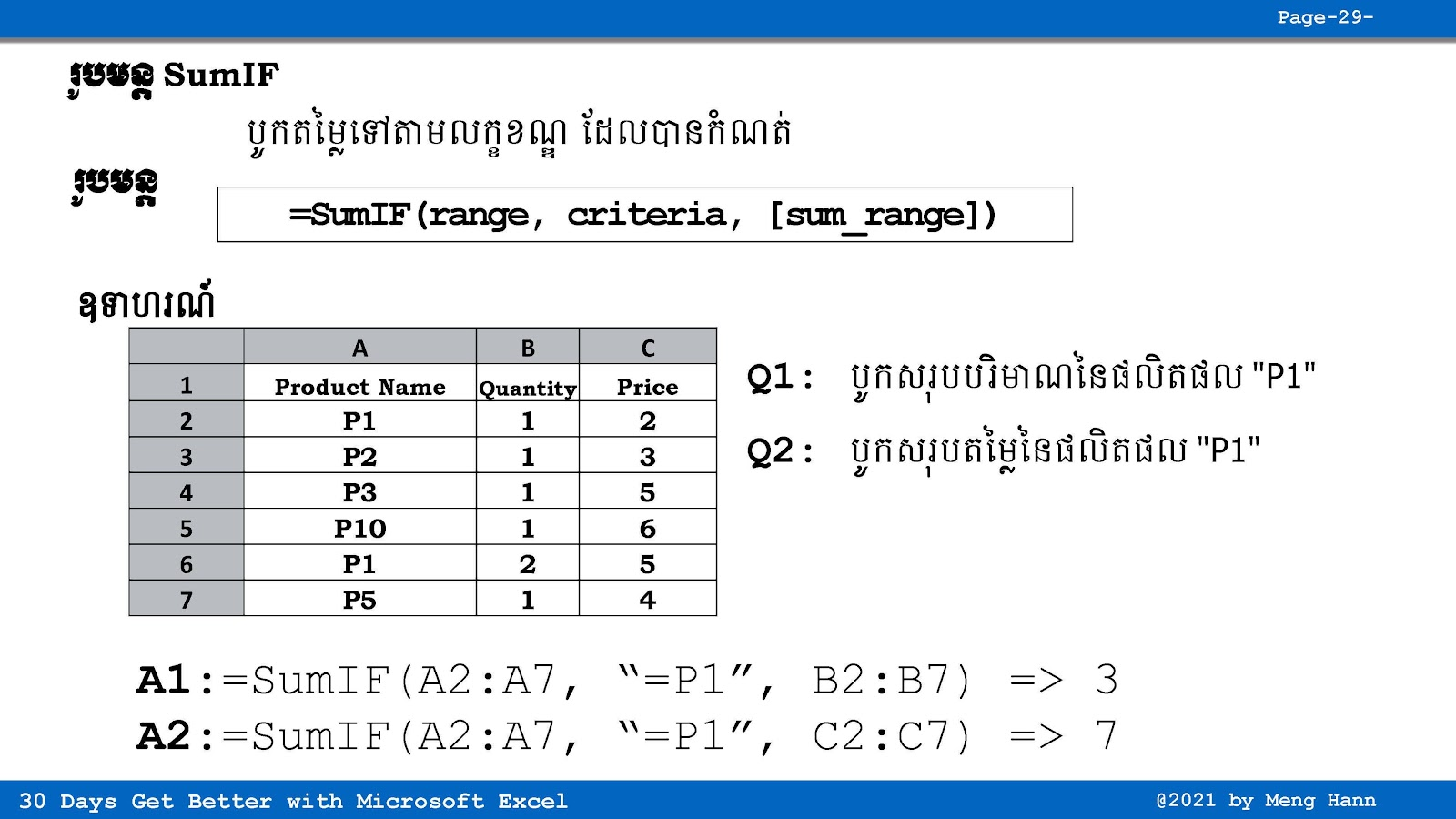# 29. How to use SUMIF formula in Excel speak Khmer

29. How to use SUMIF formula in Excel speak Khmer
If you want find total value base on certain condition, you can use SUMIF. SUMIF help you to calculate sum of value with criteria like "Male", Date, any value.

SUMIF Formula
=SUMIF(Range, Criteria, [sum_range])
◾ Range: Required, set of value or cells
◾ Criteria: Required, sum base on condition
◾ [sum_range]: optional, range to sum